Function Repository Resource:

# SchurMatrix

Generate the Schur matrix of a univariate polynomial

Contributed by: Jan Mangaldan
 ResourceFunction["SchurMatrix"][poly,x] gives the Schur matrix of poly, treated as a polynomial in x.

## Details

The Schur matrix is also called the Schur-Cohn matrix or Schur-Cohn-Fujiwara matrix.
The Schur matrix is a matrix constructed from the coefficients of a polynomial, and can be used to check if the polynomial's roots are all within the unit disk.
The Schur matrix is a Hermitian matrix.
In control theory, a polynomial is stable if all of its roots are within the unit disk. A polynomial is stable if and only if its Schur matrix is positive definite.

## Examples

### Basic Examples (1)

Generate the Schur matrix of a polynomial:

 In:=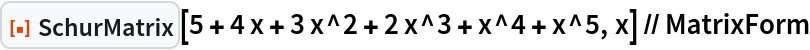Out=### Scope (3)

Generate the Schur matrix from a polynomial with numeric coefficients:

 In:=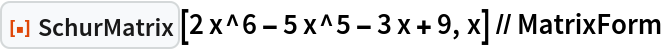Out=Generate the Schur matrix from a polynomial with symbolic coefficients:

 In:=Out=The Schur matrix for a cyclotomic polynomial is the zero matrix:

 In:=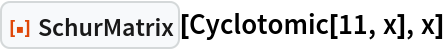Out=### Applications (2)

Use SchurMatrix to check the stability of a polynomial:

 In:=Out=In:=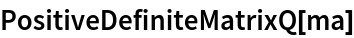Out=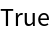Verify stability by computing the roots of the polynomial:

 In:=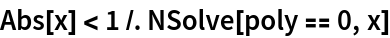Out=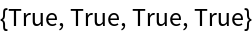The Schur matrix of a quadratic polynomial with symbolic coefficients:

 In:=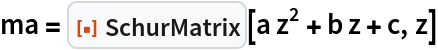Out=Use the resource function RationalCholeskyDecomposition to transform the Schur matrix into a congruent diagonal matrix:

 In:=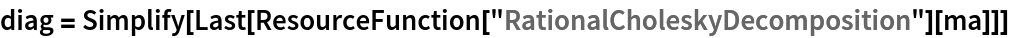Out=Determine the conditions for the Schur matrix to be positive definite (which is equivalent to the original polynomial being stable):

 In:=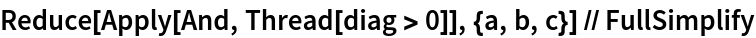Out=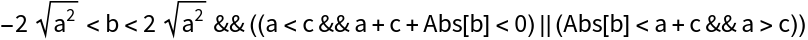### Properties and Relations (2)

The Schur matrix is Hermitian:

 In:=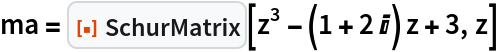Out=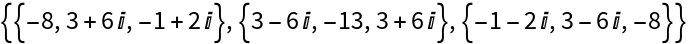In:=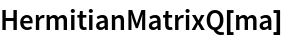Out=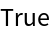The inverse of a Schur matrix is a Toeplitz matrix:

 In:=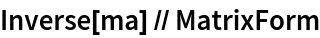Out=A polynomial whose roots are all inside the unit disk:

 In:=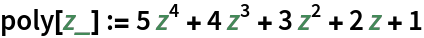The corresponding Schur matrix is positive definite:

 In:=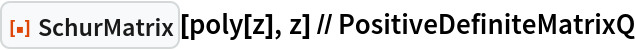Out=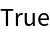Apply a Möbius transformation to the polynomial:

 In:=Out=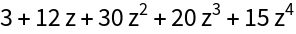The transformed polynomial has a Hurwitz matrix whose principal minors are all positive. That is, all of the roots of the transformed polynomial have negative real parts:

 In:=Out=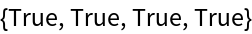## Version History

• 1.0.0 – 27 March 2023